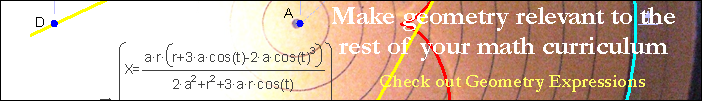TrianglesAcute TriangleAll angles are less than 90 degrees.CircumcenterThe circumcenter is the intersection of the perpendicular bisectors of the sides. It is equidistant from all of the vertices. Note that the circumcenter may also be outside or on the triangle.Equilateral TriangleAll angles and sides are equal.Isoceles TriangleAt least two sides are equal.Obtuse TriangleOne angle is greater than 90 degrees.Right TriangleOne angle is 90 degrees.Scalene TriangleAll three sides are unequal.CentroidThe centroid is the intersection of all the medians. The centroid divides all of the medians in a ratio of 2:1.OrthocenterThe orthocenter is the intersection of the triangle's altitudes. An altitude is like a height of the triangle; it is perpendicular to a side and goes through the opposite vertex.Euler LineThe orthocenter, circumcenter, and centroid always lie on the same line. This line is called the Euler Line.SSS CongruenceIf two triangles have three congruent sides, then the triangles are congruent.SAS CongruenceIf two triangles have two congruent sides and a congruent included angle, then the triangles are congruent.45-45-90 TriangleTrigonometric RatiosSAA CongruenceIf two triangles have two congruent angles and a congruent nonincluded side, then the two triangles are congruent.SSA NoncongruenceIf two triangles have two congruent sides and a congruent nonincluded angle, then they are not necessarily congruent.AAA NoncongruenceIf two triangles have three congruent angles, then they are not necessarily congruent. However, they will be similar.Side Angle SideTriangle with side angle side markedSide side sideTriangle with side-side-side markedAngle side angle (style 2)Triangle with angle-side-angle markedAngle angle sideTriangle with angle-angle-side markedAAA TriangleThis triangle shows markings for Angle-Angle-Angle. This is not a valid argument for congruence, but it does show similarity.Triangle with HeightAn obtuse triangle with extended side is shown, but the diagram can be modified to form right and acute triangles as well.30-60-90 Triangle30-60-90 triangles are sometimes called half-equilateral triangles.